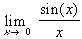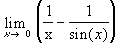#Interactive Real Analysis

## 6. Limits, Continuity, and Differentiation

### 6.5. Differentiable Functions

Having discussed continuity we will turn to another class of functions: differentiable functions. This group of functions is one of the focus points of Calculus, and you should already be familiar with many aspects of those functions

In our setting these functions will play a rather minor role and we will only briefly review the main topics of that theory. As usual, proofs will be our focus point, rather than techniques of differentiation as it has been in Calculus.

 Definition 6.5.1: Derivative Let f be a function with domain D in R, and D is an open set in R. Then the derivative of f at the point c is defined as f'(c) =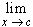If that limit exits, the function is called differentiable at c. If f is differentiable at every point in D then f is called differentiable in D. Other notations for the derivative of f are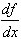or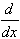f(x).
The usual geometric interpretation of the derivative at a point is as slope of the tangent line to the graph of f(x) at the point (c, f(c)). If a function is differentiable, it may not have any 'edges'. That often makes it easy to decide whether a function is differentiable if you can see the graph of the function.
 Examples 6.5.2:Find the derivative of f(x) = x and of f(x) = 1 / x.Find the derivative of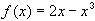Find the derivative ofAnother way to define a differentiable function is by saying that f(x) can be approximated by a linear function, as in the following theorem:
 Theorem 6.5.3: Derivative as Linear Approximation Let f be a function defined on (a, b) and c any number in (a, b). Then f is differentiable at c if and only if there exists a constant M such that f(x) = f(c) + M ( x - c ) + r(x) where the remainder function r(x) satisfies the condition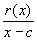= 0
This theorem provides a suitable method to generalize the concept of derivative to other spaces: a function defined in some general space is called differentiable at a point c if it can be approximated by a linear function at that point. On the real line the linear function M ( x - c ) + f(c), of course, is the equation of the tangent line to f at the point c. In higher dimensional real space this concept is known as the total derivative of a function.
 Examples 6.5.4:Why might our original definition of differentiability not be suitable for functions of, say, two or three real variables ?Use the characterization of differentiability via approximation by linear functions to define the concept of 'derivative' for functions of n real variables.
In any case, differentiability is a new concept, so that we should first ask ourselves what its relation to the previous concept of continuity is.
 Theorem 6.5.5: Differentiable and Continuity If f is differentiable at a point c, then f is continuous at that point c. The converse is not true.
 Examples 6.5.6:The function f(x) = | x | is continuous everywhere. Is it also differentiable everywhere ?The function f(x) = x sin(1/x) is continuous everywhere except at x = 0, where it has a removable discontinuity. If the function is extended appropriately to be continuous at x = 0, is it then differentiable at x = 0 ?The function f(x) = x2 sin(1/x) has a removable discontinuity at x = 0. If the function is extended appropriately to be continuous at x = 0, is it then differentiable at x = 0 ?
As with continuous functions, differentiable functions can be added, multiplied, divided, and composed with each other to yield again differentiable functions. In fact, there are easy rules to compute the derivative of those new functions, all of which are well- known from Calculus.
 Theorem 6.5.7: Algebra with Derivatives Addition Rule: If f and g are differentiable at x = c then f(x) + g(x) is differentiable at x = c, and(f(x) + g(x)) = f'(x) + g'(x) Product Rule: If f and g are differentiable at x = c then f(x) g(x) is differentiable at x = c, and(f(x) g(x)) = f'(x) g(x) + f(x) g'(x) Quotient Rule: If f and g are differentiable at x = c, and g(c) # 0 then then f(x) / g(x) is differentiable at x = c, and( f(x) / g(x) ) =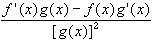Chain Rule: If g is differentiable at x = c, and f is differentiable at x = g(c) then f(g(x)) is differentiable at x = c, andf(g(x)) = f'(g(x)) g'(x)
Next, we will state several important theorems for differentiable functions:
 Theorem 6.5.8: Rolle Theorem If f is continuous on [a, b] and differentiable on (a, b), and f(a) = f(b) = 0, then there exists a number x in (a, b) such that f'(x) = 0.
An extension of Rolle's theorem that removes the conditions on f(a) and f(b) is the Mean- Value-Theorem. It is actually a 'shifted' version of Rolle's theorem, as its proof illustrates. A more general version of the Mean Value theorem is also mentioned which is sometimes useful.
 Theorem 6.5.9: Mean Value Theorem If f is continuous on [a, b] and differentiable on (a, b), then there exists a number c in (a, b) such that f'(c) =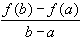If f and g are continuous on [a, b] and differentiable on (a, b) and g'(x) # 0 in (a, b) then there exists a number c in (a, b) such that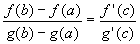Examples 6.5.10:Does Rolle's theorem apply todefined on (-3, 3) ? If so, find the number guarantied by the theorem to exist.Prove that if f is differentiable on R and | f'(x) |M for all x, then | f(x) - f(y) |M | x - y | for all numbers x, y. Functions that satisfy such an inequality are called Lipschitz functions.Use the Mean Value theorem to show that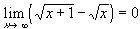Rolle's theorem and the Mean Value theorem allow us to develop the familiar test for local extrema of a function, as well as increasing and decreasing functions. Recall the definition of local extremum:
 Definition 6.5.11: Local Extremum Let f be a function defined on a domain D, and c a point in D. If there exists a neighborhood U of c with f(c)f(x) for all x in U, then f(c) is called a local maximum for the function f that occurs at x = c. If there exists a neighborhood U of c with f(c)f(x) for all x in U, then f(c) is called a local minimum for the function f that occurs at x = c. If f(x) has either a local minimum or a local maximum at x = c, then f(c) is called local extremum of the function f.
You can find possible local extrema by applying the following theorem:
 Theorem 6.5.12: Local Extrema and Monotonicity If f is differentiable on (a, b), and f has a local extrema at x = c, then f'(c) = 0. If f'(x) > 0 on (a, b) then f is increasing on (a, b). If f'(x) < 0 on (a, b) then f is decreasing on (a, b).
This theorem suggests the following table in order to find local minima and maxima: Suppose you have found a point c such that f'(c) either does not exist or f'(c) = 0. For each c (called a critical point of f) we may have one of these four situations:
 Loc. Max interval (a, c) (c , b) sign of f'(x) + - dir. of f(x) up down
 Loc. Min interval (a, c) (c , b) sign of f'(x) - + dir. of f(x) down up
 No Extremum interval (a, c) (c , b) sign of f'(x) + + dir. of f(x) up up
 No Extremum interval (a, c) (c , b) sign of f'(x) - - dir. of f(x) down down
The results of these tables can be summarized in the following:
 Corollary 6.5.13: Finding Local Extrema Suppose f is differentiable on (a, b). Then: If f'(c) = 0 and f'(x) > 0 on (a, x) and f'(x) < 0 on (x, b), then f(c) is a local maximum. If f'(c) = 0 and f'(x) < 0 on (a, x) and f'(x) > 0 on (x, b), then f(c) is a local minimum.
These results above are the cornerstones of Calculus 1 in most colleges. As a review, you may enjoy the following examples:
 Examples 6.5.14:If f(x) = x3 -2 x2, then find all local extrema.If f(x) = | 1 - x2|, then find all relative extrema.
One of the nice applications of derivatives is that they give an easy short-cut rule to finding limits, when those limits are difficult to obtain otherwise.
 Theorem 6.5.15: l Hospital Rules If f and g are differentiable in a neighborhood of x = c, and f(c) = g(c) = 0, then, provided the limit on the right exists. The same result holds for one-sided limits. If f and g are differentiable and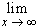f(x) =g(x) =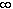,then, provided the last limit exists

There are other situations where l'Hospital's rule may apply, but often expressions can be rewritten so that one of these two cases will apply.

 Examples 6.5.16: Please find the following limits, using, if necessary, l'Hospital's rules: